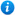# Addition and Subtraction are Opposites - Year 1

Addition and Subtraction are Opposites - Year 1

Year 1 maths programme of study - Number (addition and subtraction):

• represent and use number bonds and related subtraction facts within 20
• add and subtract one-digit and two-digit numbers to 20

This KS1 maths teaching resource introduces children to the inverse relationship between addition and subtraction while practising adding and subtracting within 20 as per the year 1 maths programme of study (Number - addition and subtraction).

This colourful animated PowerPoint presentation includes

• What are opposites explanation
• An explanation of why addition and subtraction are opposites
• 1 activity with accompanying worksheet
• 1 further worksheet with answers

'Addition and Subtraction are Opposites - Year 1' can be edited allowing teachers to adapt the resource, if they wish, to suit the individual needs of each class they teach.

To take a look at 'Addition and Subtraction are Opposites - Year 1' please click on the images from the PowerPoint lesson.

Our Price : £1.99 / 2 Credits## Get this resource as part of a bundle and save up to 45%## Year 1 Addition and Subtraction Bundle

£8.99

By continuing to use the site, you agree to the use of cookies. You can change this and find out more by following this link